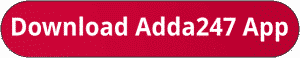Latest Banking jobs   »   bank exam 2023   »   bank exam 2023

# Quantitative Aptitude Quiz For Bank Mains Exam 2023-02nd November

Directions (1-3): The following questions are accompanied by two statements I and II. You have to determine which statements(s) is/are sufficient/necessary to answer the questions.

Q1. Is Rahul meet Prabhat at half of the distance on a circular track (48km) if they both started to move at same time in same direction?
I. Speed of Prabhat is 24km/hr more than speed of Rahul.
II. Prabhat’s speed is 200% more than that of Rahul
(a) Statement I alone is sufficient to answer the question but statement II alone is not sufficient to answer the question.
(b) Either statement I or statement II by itself is sufficient to answer the question.
(c) Both the statements taken together are necessary to answer the questions, but neither of the statements alone is sufficient to answer the question.
(d) Statement II alone is sufficient to answer the question but statement I alone is not sufficient to answer the question.
(e) Statements I and II taken together are not sufficient to answer the question.

Q2. Find number of yellow balls in a box which contains 3 black balls and 7 red and yellow balls.
I. Probability of selecting two yellow balls from the box is 2/15
II. Probability of selecting one red and black ball is 1/5.
(a) Statement I alone is sufficient to answer the question but statement II alone is not sufficient to answer the question.
(b) Statement II alone is sufficient to answer the question but statement I alone is not sufficient to answer the question.
(c) Both the statements taken together are necessary to answer the questions, but neither of the statements alone is sufficient to answer the question.
(d) Either statement I or statement II by itself is sufficient to answer the question.
(e) Statements I and II taken together are not sufficient to answer the question.

Q3. Find number of pens sold by retailer if he earns total 37.5% profit on selling some pens and some pencils.
I. Ratio between pen sold to pencil sold is 2 : 3 while ratio between cost price of pen to pencil is 3 : 2.
II. On selling a pen and a pencil, he earns 25% and 50% profit respectively.
(a) Both the statements taken together are necessary to answer the questions, but neither of the statements alone is sufficient to answer the question.
(b) Statements I and II taken together are not sufficient to answer the question.
(c) Statement II alone is sufficient to answer the question but statement I alone is not sufficient to answer the question.
(d) Statement I alone is sufficient to answer the question but statement II alone is not sufficient to answer the question.
(e) Either statement I or statement II by itself is sufficient to answer the question.

Directions (4-5): The following questions are accompanied by two statements (I) and (II). You have to determine which statement(s) is/are sufficient / necessary to answer the questions.
Q4. Ratio between radius and height of a right circular cylinder is 3 : 2. Find area of a square?
I . Given that side of square is equal to the radius of cylinder.
II . Height of cylinder is equal to the radius of circle whose area is 616 cm2.
(a) Only statement I is sufficient
(b) Only statement II is sufficient
(c) Statement I and II both
(d) Either statement I or Statement II alone sufficient
(e) Neither statement I nor statement II sufficient

Q5. Ratio between length of two trains is 7 : 6. What will be difference between lengths of both trains?
I . Speed of smaller train is 108 km/hr and it can cross an unmoving man in 2.7 sec.
II . Time taken by larger train to cross the smaller train is 5.4 sec and speed of larger train is 90 km/hr.
(a) Only statement I is sufficient
(b) Only statement II is sufficient
(c) Statement I and II both
(d) Either statement I or Statement II alone sufficient
(e) Neither statement I nor statement II sufficient

Solutions.                                                           .                                                    .

## FAQs

### When is the IBPS Clerk Mains exam scheduled for?

The IBPS Clerk Mains exam is scheduled for 7 October 2023.

#### Congratulations!Union Budget 2023-24: Free PDF